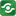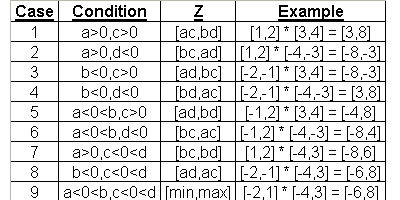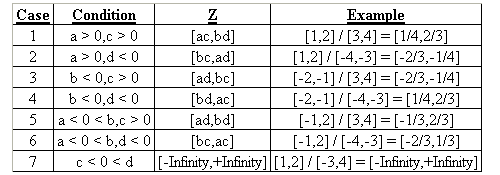Interval Calculator@import url(http://www.google.com/cse/api/branding.css);CSGNetwork Custom SearchCSGNetwork Snippets Calculators Top Free Apps Home Back

Right click this window and select "view source" in order to copy the source for this script.

Interval Calculator

 X: [ , ] Y: [ , ] Result: [ , ]

 Input: Expressions:

 MID: MIG: MAG: WID:

How the operations work

Adding Intervals together is rather easy. To add Intervals together simply add the lowest X value together with the lowest Y value, then add the highest X value with the highest Y value.

X Values: [a,b]
Y Values: [c,d]

When calculating Intervals the X values are placed "on top of" the Y values. Also the lowest value of either the X of the Y is placed first in the set. When adding you are adding a+c and b+d.

X Values: [a,b]
Y Values: + [c,d]
Result: [e,f]

[1,3]
+ [2,4]
[3,7]

Subtraction

Subtracting Intervals is much like adding Intervals. To subtract Intervals simply subtract the lowest X value by the highest Y value, then subtract the highest X value by the lowest Y value.

X Values: [a,b]
Y Values: [c,d]
When calculating Intervals the X values are placed "on top of" the Y values. Also the lowest value of either the X of the Y is placed first in the set. When subtracting you are subtracting a-d and b-c.

X Values: [a,b]
Y Values: - [c,d]
Result: [e,f]
[1,3]
- [2,4]
[-3,1]

Multiplication

Multiplying Intervals is a lot more complicated then adding and subtracting Intervals. To multiply Intervals follow the steps below:

low X=a
high X=b
low Y=c
high Y=d
low result=e
high result=f

A) If a >= 0
1. If c >= 0
a) a * c = e
b) b * d = f

2. If d <= 0
a) b * c = e
b) a * d = f

3. If neither 1 or 2 apply
a) b * c = e
b) b * d = e

B) If b <= 0
1. If c >= 0
a) a * d = e
b) b * c = f

2. If d <= 0
a) b * d = e
b) a * c = f
3. If neither 1 of 2 apply
a) a * d = e
b) a * c = f

C) If neither A of B apply
1. If c >= 0
a) a * d = e
b) b * d = f
2. If d <= 0
a) b * c = e
b) a * c = f

3. If neither 1 or 2 apply
a) e = Minimum of (a * d, b * c)
b) f = Maximum of (a * c, b * d)

X Values: [a,b]
Y Values: [c,d]

When calculating Intervals the X values are placed "on top of" the Y values. Also the lowest value of either the X of the Y is placed first in the set.

X Values: [a,b]
Y Values: * [c,d]
Result: [e,f]

[1,3]
* [2,4]
[2,12]Division

Dividing Intervals is a lot more complicated then adding and subtracting Intervals. To divide Intervals follow the steps below:

low X=a
high X=b
low Y=c
high Y=d
low result=e
high result=f

A) If c >= 0
1. If a >= 0
a) a / d = e
b) b / c = f

2. If b <= 0
a) a / c = e
b) b / d = f

3. If neither 1 or 2 apply
a) a / c = e
b) b / c = e
B) If d <= 0
1. If a >= 0
a) b / d = e
b) a / c = f

2. If b <= 0
a) b / c = e
b) a / d = f

3. If neither 1 of 2 apply
a) b / d = e
b) a / d = f

C) If c <= 0 and d >= 0
1. If c >= 0
a) e = -INFINITY
b) f = +INFINITY

X Values: [a,b]
Y Values: [c,d]

When calculating Intervals the X values are placed "on top of" the Y values. Also the lowest value of either the X of the Y is placed first in the set.

X Values: [a,b]
Y Values: / [c,d]
Result: [e,f]

[1,3]
/ [2,4]
[.25,1.5]The calculator performs interval arithmetic operations and computers interval version of mathematical functions. It is an ongoing work.

Definitions of Input Boxes:
Input: - is the box where numbers are entered into the calculator
Expressions: - is the box where expression like a+b are inputed

Definitions of Buttons:
Compute - computes the expression inputed in the Expressions box
ClrX - clears the top set (x) of intervals
ClrY - clears the bottom set (y) of intervals
Enter - places the value in the Input Box into the next available spot
AC - Clears all values
Top - places the result into the X boxes
+/- - used to input negative numbers
E - used to input scientific notation
C - clears the Input Box
^ - raise the X values by the power of Y
NegX - switches the X values and turns them into the opposite value
NegY - switches the Y values and turns them into the opposite value
log - computes the common log of the X values
sqrt - computes the square roots of the X values
1/x - reciprocal of the X values
1/y - reciprocal of the Y values
e^X - raises e by the power of the X values
ln - computes the natural log to the X values
max - finds the maximum range
min - finds the minimum range
ABS - finds the absolute values
IX - the intercept point
IH - the convex hull

View the source...